Tetrahedral hard sphere model

The tetrahedral hard sphere model consists of four hard spheres located on the vertices of a regular tetrahedron.

Second virial coefficient

The second virial coefficient is given by ( Eq.5):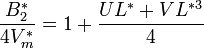$\frac{B_2^*}{4V_m^*} = 1 + \frac{UL^* + VL^{*3}}{4}$

where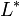$L^*$ is the reduced elongation,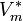$V_m^*$ is the corresponding reduced volume,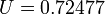$U=0.72477$ and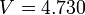$V=4.730$.

Equation of state

The equation of state is given by ( Eq. 17):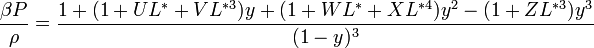$\frac{\beta P}{\rho} = \frac{1+(1+UL^* + VL^{*3})y + (1+WL^* + XL^{*4})y^2 - (1+ ZL^{*3})y^3}{(1-y)^3}$

where$U=0.72477$,$V=4.730$,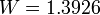$W=1.3926$,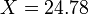$X=24.78$ and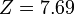$Z=7.69$.